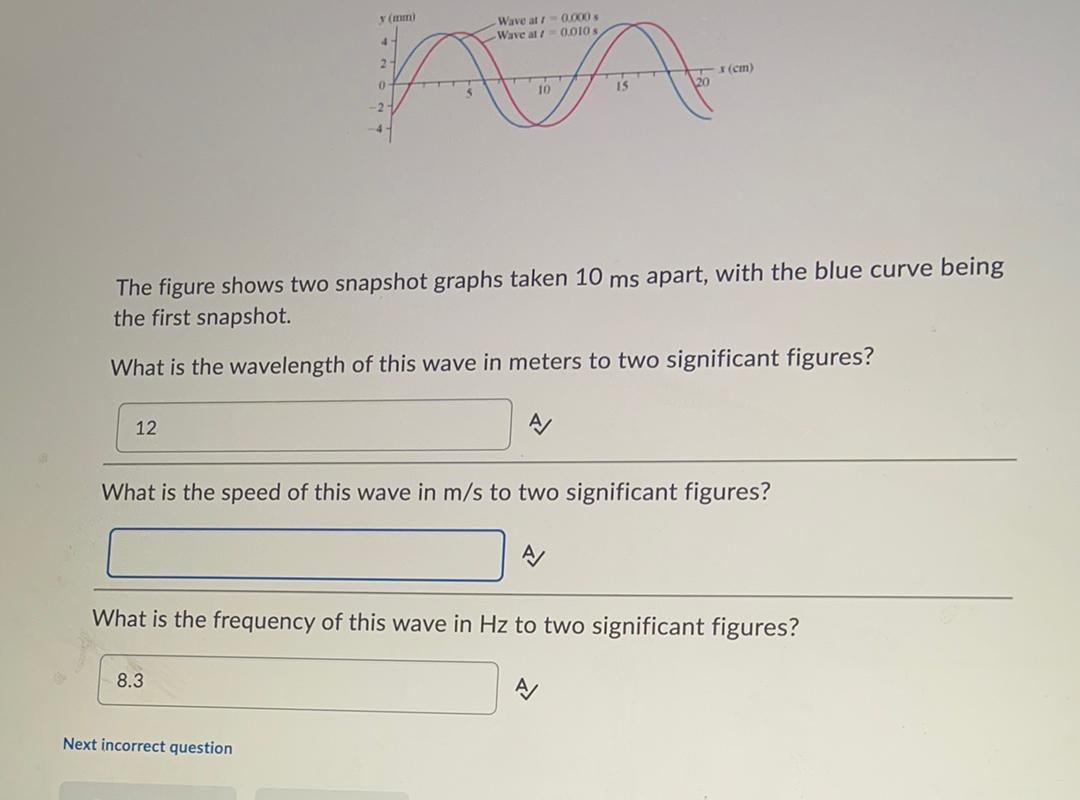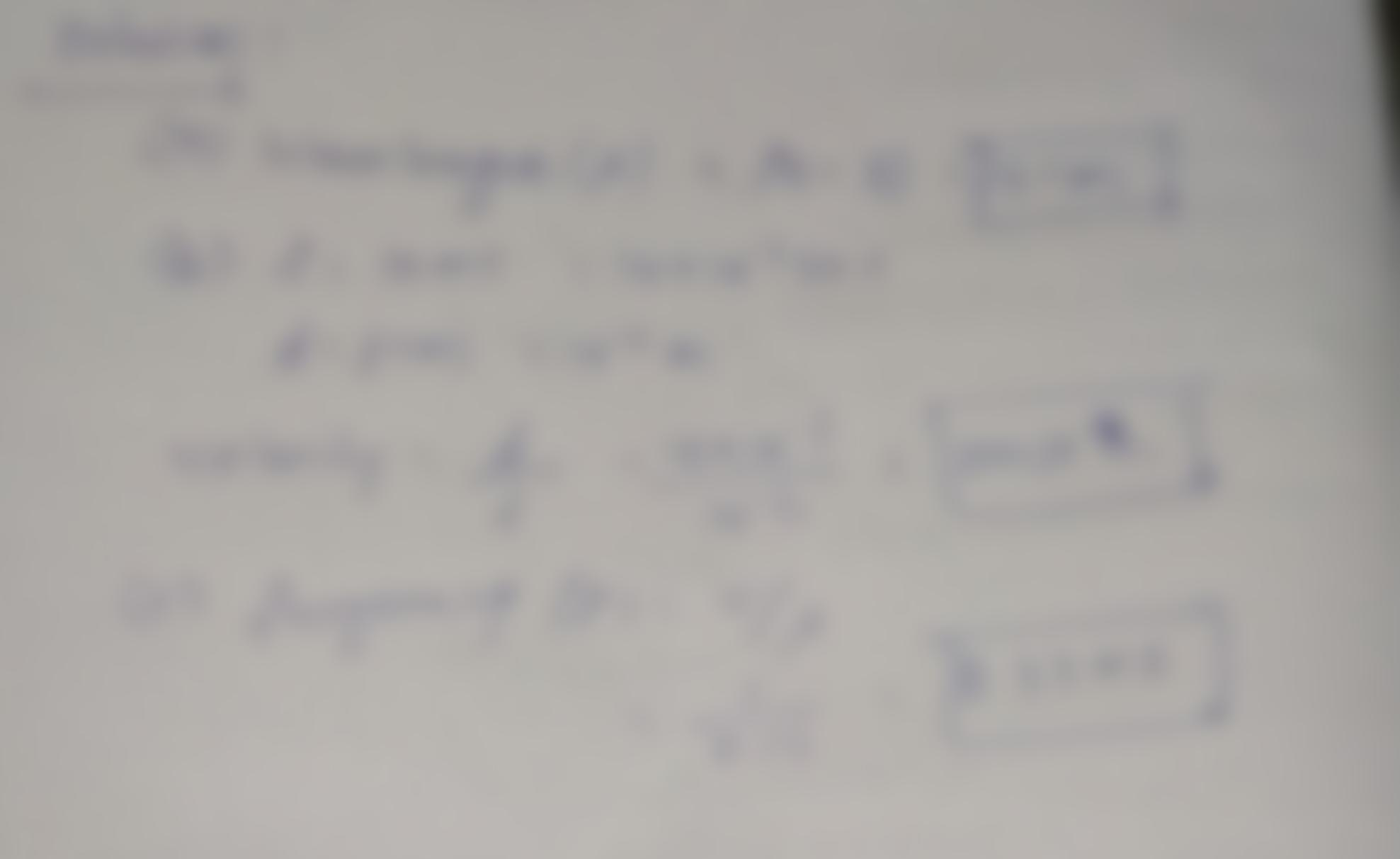Question:

(mm) Wave at 0.000 -Wave all 0.010s 4 2 (cm) 0 10 15 2 The figure shows two snapshot graphs taken 10 ms apart, with the blue cur(mm) Wave at 0.000 -Wave all 0.010s 4 2 (cm) 0 10 15 2 The figure shows two snapshot graphs taken 10 ms apart, with the blue curve being the first snapshot. What is the wavelength of this wave in meters to two significant figures? 12 What is the speed of this wave in m/s to two significant figures? What is the frequency of this wave in Hz to two significant figures? 8.3 A Next incorrect question